# Fibonacci Numbers Spelled Out

by Ivan Galkin,
U.MASS Lowell
Materials for 91.503 Algorithms

 "Spelled Out" series contains all derivations usually omitted by gurus and left for the reader to agonize over. Spelled out documents are written in a free style to ease the pain.

### 1. Fibonacci Numbers, or "How many rabbits will you get after a year?"

Here they are:

0, 1, 1, 2, 3, 5, 8, 13, 21, 34, ...

each number is a sum of two previous.

We can write a difference equation for Fibonacci numbers as: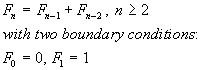(1)

### 2. Generating Function Approach

A useful way to describe an integer sequence is to construct a generating function B(x):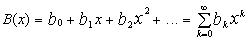(2)
whose coefficients bi are the sequence. B(x) is a power series over a purely formal variable x. ("Power" series just indicates that the powers of x are used in the series.)

The whole point of building B(x) is to figure out an analytic formula for those bi. The usual trick is to find a closed form expression for B(x) and tweak it.

#### 2.1 Closed-form expression

Some power series are able to converge to an expression which does not include infinite summation anymore. For instance, an infinite sequence of the numbers (dear to our hearts):
1, 2, 4, 8, 16, 32, 64, 128, 256 ...
can be represented by a generating function: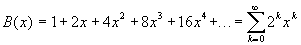which can be, suprisingly, put in a closed form: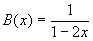For this to happen, x must be less than 1 -- otherwise the sum terms grow and it can not converge.
Just to give us a feeling, let's select x = 0.1: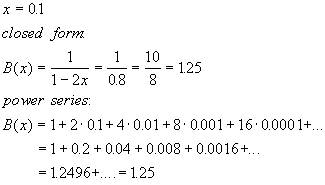It works!
 A popular closed form expression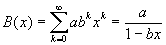(3)

#### 2.2 Closed-form Expression for Fibonacci Numbers' Generating Function

So the idea is to assume a generic generating function (2) with coefficients representing the Fibonacci numbers. Now we have to find some regularity in the behavior of the coefficients.

Here's the trick:(4)
And this is a closed-form expression for the Fibonacci numbers' generating function.

The point here is that generating function turns the recursive equation (1) with two boundary conditions into something more managable.And it is because it can kinda transform (n-1) terms into xB(x), (n-2) into x2B(x), etc.

#### 2.4. Verifying Closed-Form Expression for Fibonacci #s GF by Long Division

Well, I studied "stolbikom" division in USSR, so I apologize if my arrangement on the paper look confusing to you. It sure does to me. Check here for a polynomial division example I used to figure out US notation.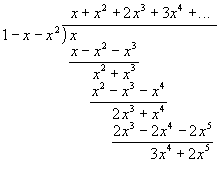The point is -- here's our sequence 1, 1, 2, 3, ...   er, where do I look for the answer, again? Oh well, the top line. Now we see how GFs work -- if we expand them in series over xn, the original sequence is generated.

#### 2.5. Expanding Closed-form Expression for GF

The next step is to find a good (closed-form) expression for k-th Fibonacci number.  The basic idea is to expand the closed-form expression for GF (4) back to the infinite power series. Well, there is a generic way of expanding a ratio of polynomials into Taylor series around the poles. Instead, we can use (courtesy of Bernulli) algebraic manipulation which shall reduce (4) into a form of some known generating function expansion (like (3)):We shall find these a and b for the case of Fibonacci GF (4).

1. First, we have to split quadratic polynomial in the denominator of (4):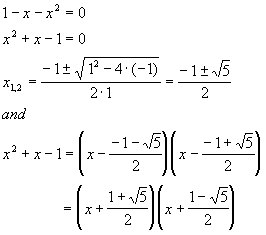2. It really helps at this point to introduce a "Golden Section" or "Divine Proportion", F: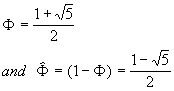After that our generating function becomes: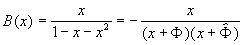This is not good enough: we need constructions ( 1 - bx ) in the denominator. So our next trick is:

3.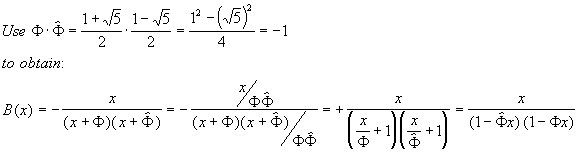4. Now, we split the fraction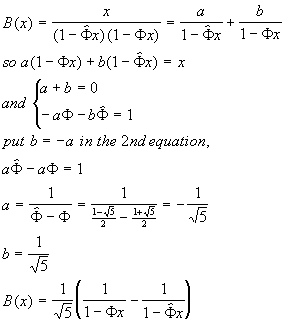(6)
5. And, finally, we compare (6) to (3) to conclude: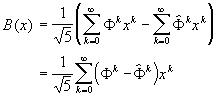Here are our Fibonacci numbers:
 Fibonacci Numbers, closed-form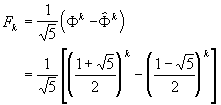(7)

## 3. Characteristic Polynomial Approach

Another techcnique of solving difference equation (1) is to let Fn to be a characteristic polynomial rn, which appears to quickly reduce (1) to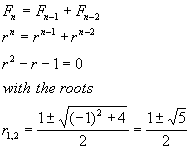These roots are different from what we had in Section 2.The general solution to equation Fnrn will be all possible combinations of roots r1 and r2: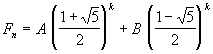Constants A and B can be found from the boundary conditions F0=0 and F1=1: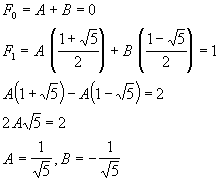So we arrive at familiar expression for Fibonacci numbers (7).